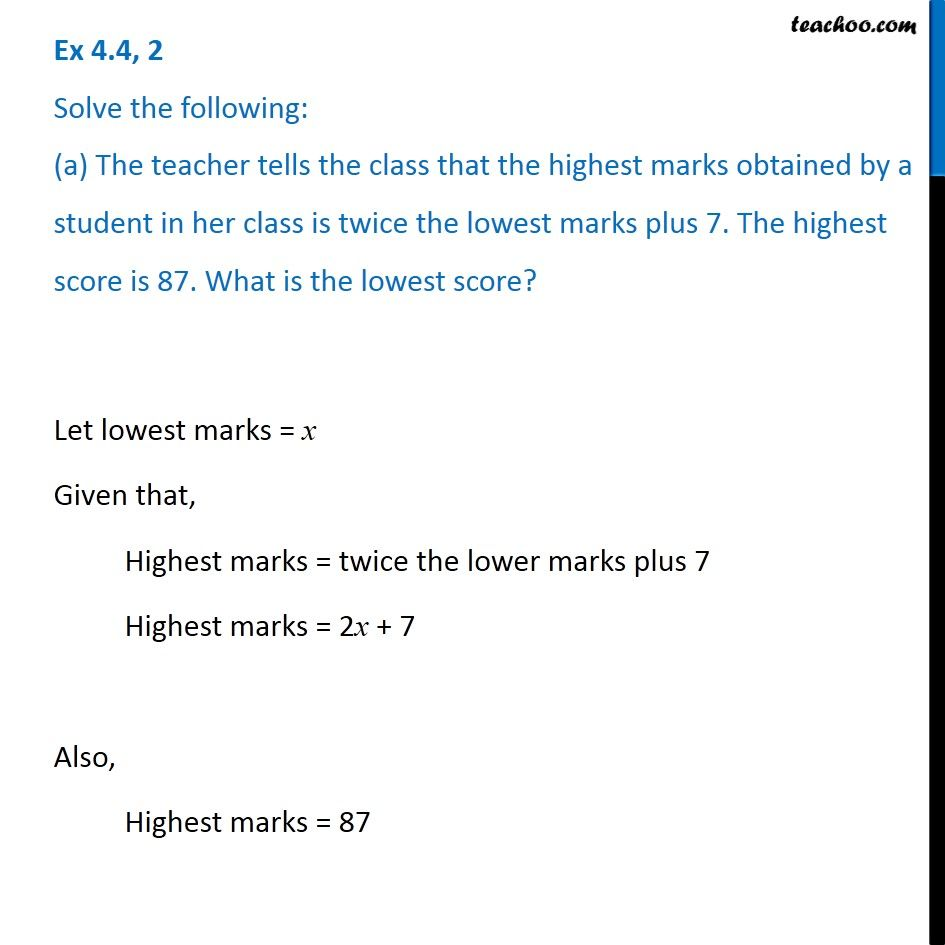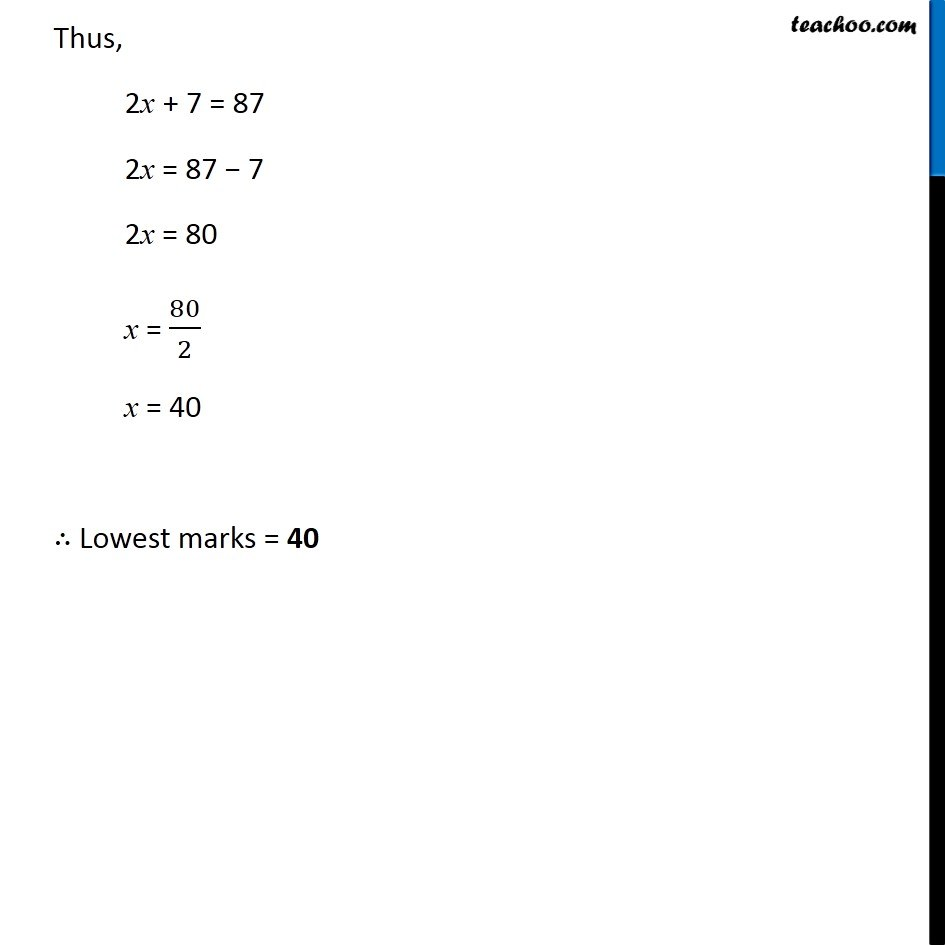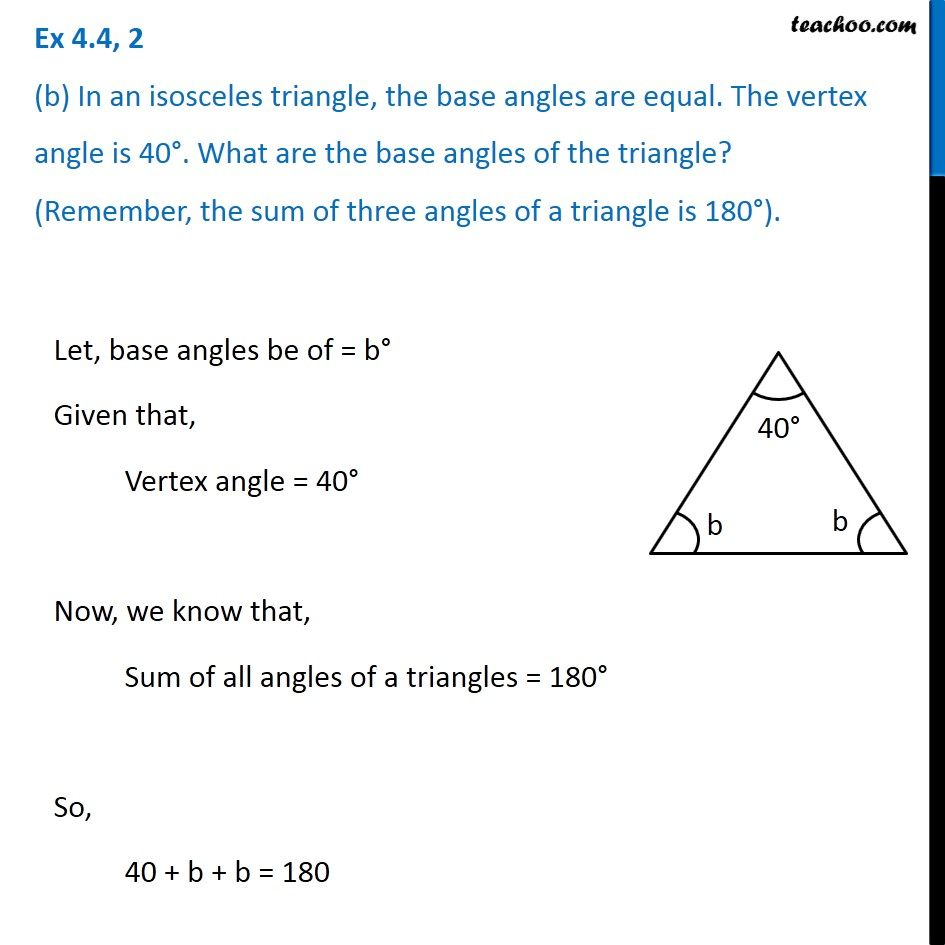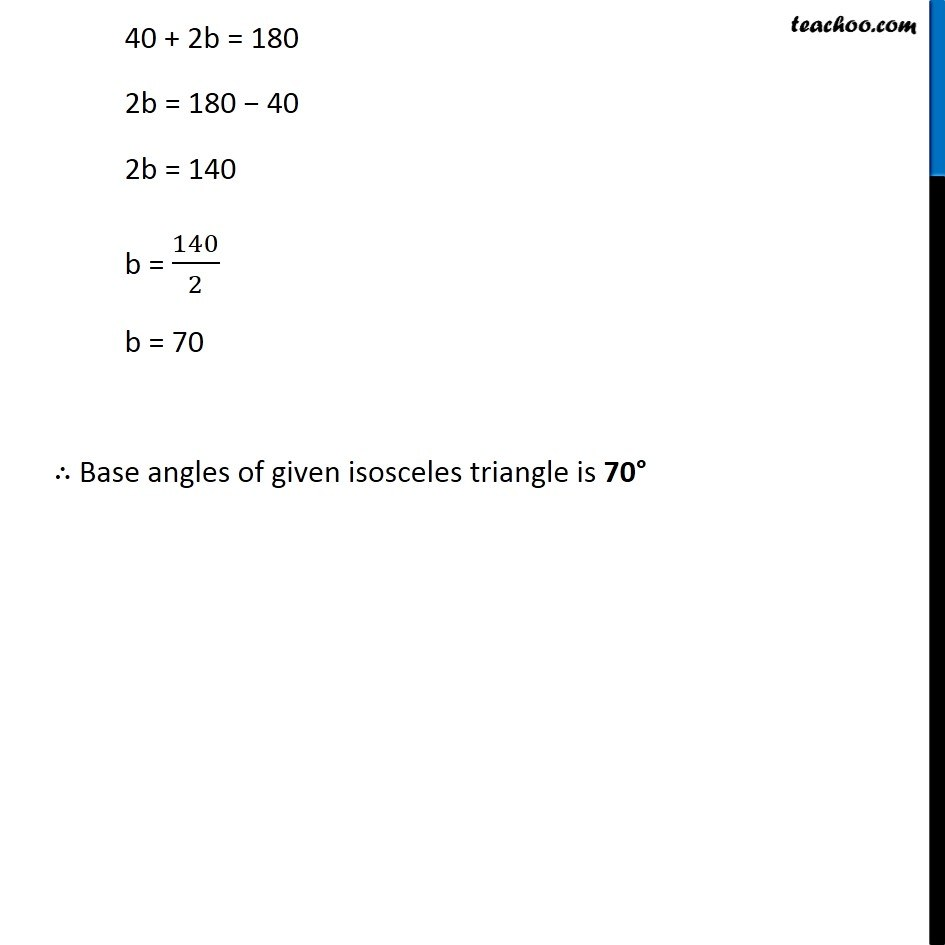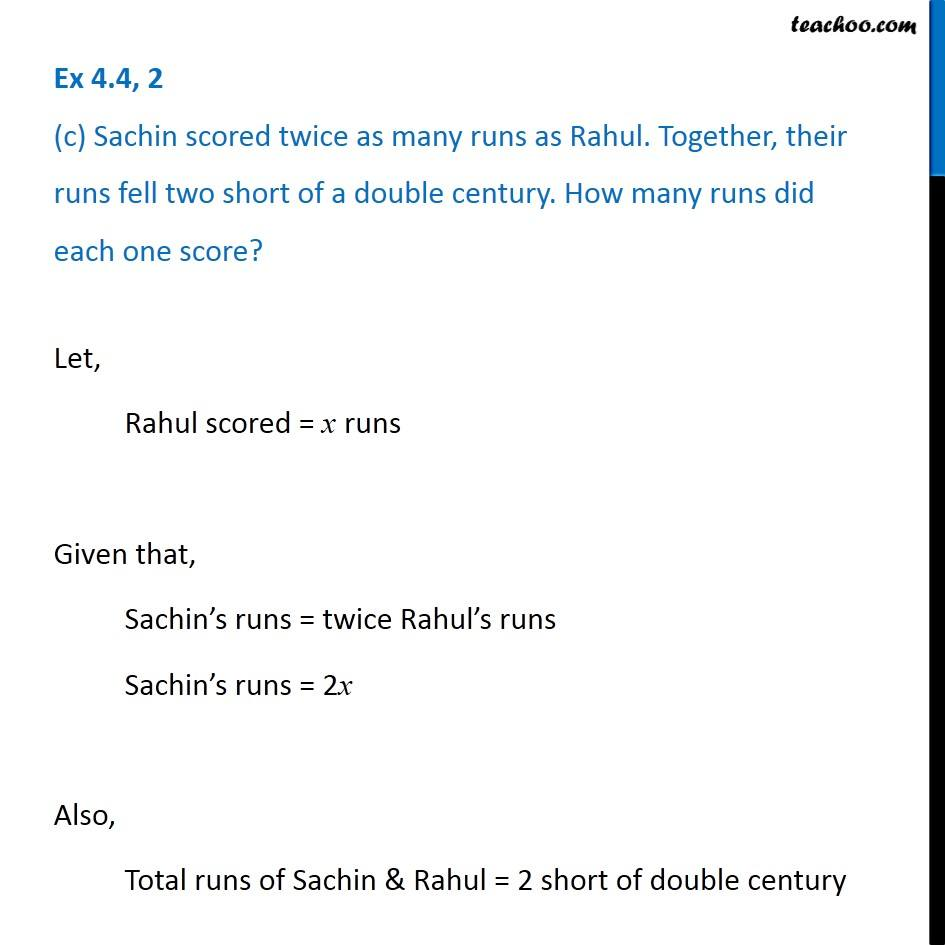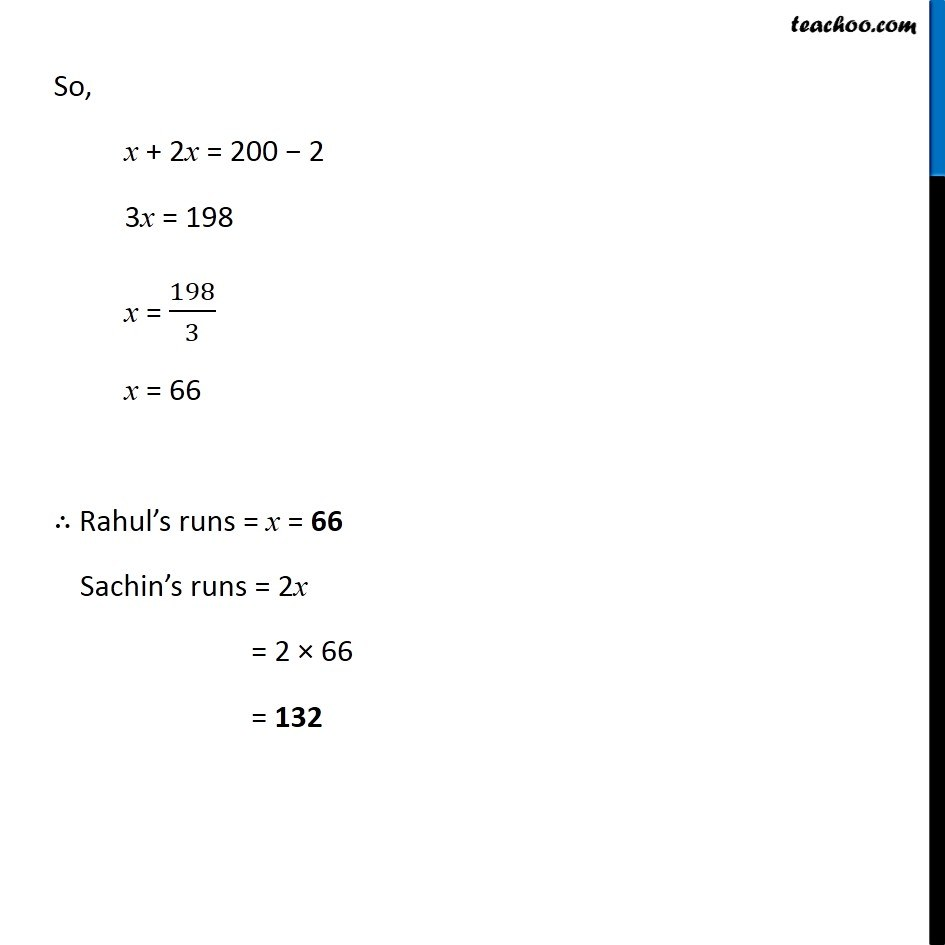Subscribe to our Youtube Channel - https://you.tube/teachoo

1. Chapter 4 Class 7 Simple Equations
2. Serial order wise
3. Ex 4.4

Transcript

Ex 4.4, 2 Solve the following: (a) The teacher tells the class that the highest marks obtained by a student in her class is twice the lowest marks plus 7. The highest score is 87. What is the lowest score?Let lowest marks = x Given that, Highest marks = twice the lower marks plus 7 Highest marks = 2x + 7 Also, Highest marks = 87 Thus, 2x + 7 = 87 2x = 87 − 7 2x = 80 x = 80/2 x = 40 ∴ Lowest marks = 40 Ex 4.4, 2 (b) In an isosceles triangle, the base angles are equal. The vertex angle is 40°. What are the base angles of the triangle? (Remember, the sum of three angles of a triangle is 180°).Let, base angles be of = b° Given that, Vertex angle = 40° Now, we know that, Sum of all angles of a triangles = 180° So, 40 + b + b = 180 40 + 2b = 180 2b = 180 − 40 2b = 140 b = 140/2 b = 70 ∴ Base angles of given isosceles triangle is 70° Ex 4.4, 2 (c) Sachin scored twice as many runs as Rahul. Together, their runs fell two short of a double century. How many runs did each one score?Let, Rahul scored = x runs Given that, Sachin’s runs = twice Rahul’s runs Sachin’s runs = 2x Also, Total runs of Sachin & Rahul = 2 short of double century So, x + 2x = 200 − 2 3x = 198 x = 198/3 x = 66 ∴ Rahul’s runs = x = 66 Sachin’s runs = 2x = 2 × 66 = 132

Ex 4.4